1800-1023-196

+91-120-4616500

# Electrode Potential

## Electrode Potential

(1) When a metal (M) is placed in a solution of its ions (M++), either of the following three possibilities can occurs, according to the electrode potential solution pressure theory of Nernst.

(i) A metal ion M n+ collides with the electrode, and undergoes no change.

(ii) A metal ion M n+ collides with the electrode, gains n electrons and gets converted into a metal atom M, (i.e. the metal ion is reduced).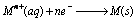(iii) A metal atom on the electrode M may lose an electrons to the electrode, and enter to the solution as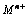, (i.e. the metal atom is oxidised).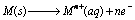.

Thus, “the electrode potential is the tendency of an electrode to lose or gain electrons when it is in contact with solution of its own ions.”

(2) The magnitude of electrode potential depends on the following factors,

(i) Nature of the electrode,

(ii) Concentration of the ions in solution,

(iii) Temperature.

(3) Types of electrode potential: Depending on the nature of the metal electrode to lose or gain electrons, the electrode potential may be of two types,

(i) Oxidation potential: When electrode is negatively charged with respect to solution, i.e., it acts as anode. Oxidation occurs.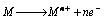(ii) Reduction potential: When electrode is positively charged with respect to solution, i.e. it acts as cathode. Reduction occurs.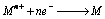(4) Standard electrode potential : “If in the half cell, the metal rod (M) is suspended in a solution of one molar concentration, and the temperature is kept at 298 K, the electrode potential is called standard electrode potential, represented usually by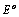”. ‘or’

The standard electrode potential of a metal may be defined as “the potential difference in volts developed in a cell consisting of two electrodes, the pure metal in contact with a molar solution of one of its ions and the normal hydrogen electrode (NHE)”.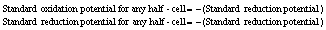(5) Reference electrode or reference half - cells

It is not possible to measure the absolute value of the single electrode potential directly. Only the difference in potential between two electrodes can be measured experimentally. It is, therefore, necessary to couple the electrode with another electrode whose potential is known. This electrode is termed as reference electrode or reference half - cells. Various types of half – cells have been used to make complete cell with spontaneous reaction in forward direction. These half – cells have been summarised in following table,

## NEET & AIIMS Exam Sample Papers

 AIIMS SAMPLE PAPERS View More NEET SAMPLE PAPERS View More